## Yenka: free maths software to use at home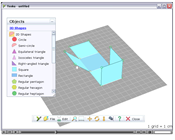More suited to older students, but well worth a mention is the new software out from Crocodile-clips called Yenka.

Yenka is a new generation of educational software.  There are 13 powerful modules which let you experiment safely with maths, science and technology in a 3D virtual world.
They have 2 products specifically for maths, Yenka Statistics and Yenka 3D Shapes. They let you experiment with concepts in a colourful 3D environment.

To help spread the word, students and teachers can get free home licences all these Yenka products – no purchase necessary! (NB The free home licences unfortunately aren’t yet available in India. This is because of an existing agreement they have with a partner there.)

Contact [email protected] or visit www.yenka.com for more information. If you are working with nets of 3D objects then certainly sign up and have a look.

## Year 3 maths worksheet: Counting on patterns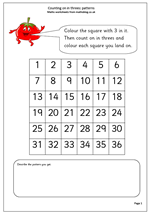Number squares can be a great way to show some of the fantastic and fascinating patterns that numbers can make. We are used to the 10 by 10 number square but, of course, they can be any size and the different sizes can create different patterns when counting on. For example, the first number square on this maths worksheet is a 6 by 6 square. When the multiples of 3 are coloured we get two vertical lines of colour. However, when using a 7 by 7 square, the pattern changes. Why is this?

This type of exercise can be used many times and soon children should be able to predict the kind of pattern that will be made, as well as having some practice with learning their tables!

Counting on in 3s patterns

## Year 4 Maths number worksheet: Half way between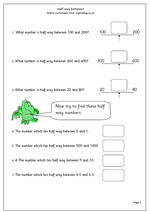Finding the number which lies half way between two other numbers can be easy or quite tricky, even when using fairly small numbers. When using whole tens or hundreds the questions tend to be quite straightforward, as does finding halfway between two consecutive whole numbers. Most of the questions on this number worksheet are of this easier type. One of the harder questions here is to find half way between 20 and 80 and it is interesting to ask how people go about this mentally. Often they will conduct a mental process of adding ten to the lower number and subtracting ten from the higher number until they meet ‘in the middle’.

## Free maths worksheet: Division and multiplication corresponding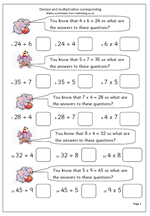This maths worksheet looks at the correspondence between multiplication and division. Given one fact, three others can be worked out. For example:

Given that  5 x 4 = 20 then:

a. 4 x 5 = 20

b. 20 ÷ 5 = 4

c. 20 ÷ 4 = 5

A full understanding of this relationship is needed before children can go on to written methods of division. If your child is struggling with division it would be well worth checking that they understand this.

To make sense of this it is a good idea to ‘talk through’ what these number sentences say.

If 5 lots of 4 are 20 then 4 lots of 5 will also be 20.

If 20 sweets are shared equally between 4 people they get 5 each.

If 20 sweets are shared equally between 5 people they get 4 each.

Multiplication and division corresponding

## Y4 maths worksheet: more or less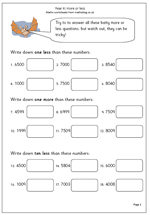Writing down one or ten more/less than a number might seem quite easy but when it means crossing a hundreds or thousands boundary it is not quite as simple as it appears. For example: ten less than 5804.

This maths worksheet on more than and less than should show whether place value has been fully understood or whether more practice needs to be done with counting on and back crossing hundreds and thousands.

It is a good idea to say the numbers out loud when carrying out this exercise.

Year 4 maths worksheet: More or less pg 1

## News: Primary children to study Twitter and blogs

Draft plans for a new Primary Curriculum for children include studying Twitter, blogging, Wiki and podcasts.

According to the Guardian today these plans, drawn up by Jim Rose are part of a new six ‘core learning areas’ that should replace the current 13 subject areas.

As far as maths goes the biggest change seems to be less emphasis on using calculators with older children, which is a shame as there is an amazing amount of good mathematical investigation that can be done with a calculator (ignoring the simplistic quick way to do a sum.).

I am not sure that studying Wiki is a worthwhile long term exercise: it ‘s very easy to use and should take about 10 minutes for children to find their way around. As for blogging, perhaps it should be made compulsory for every child to read my blogs!

More on this.

## Year 2 maths worksheet:Understanding division as sharing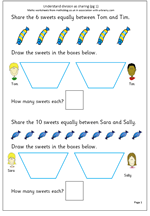Division is usually the hardest of the four rules for children to learn, but in the early stages it is quite straightforward. Children often come across division for the first time when sharing, usually between two. The key concept,of course, is that the sharing is done equally. So, for example, 6 sweets shared between 2 children implies that the sharing is equal and they both receive the same number.

By far the best way to practice sharing is to use practical apparatus or use real life situations. Eg share the strawberries equally between two, share or deal the cards equally etc. Usually this is done on a ‘one for you and one for me’ type process until there are none left. This maths worksheet replicates a practical situation, with the ultimate aim that children begin to remember the answers, which, of course are the inverse of multiplication.

Division as sharing (pg 1)

## Y2 addition worksheet: find the missing number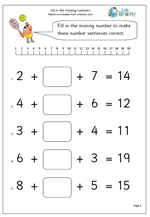Sometimes sums can prove to be more difficult than they first appear. For many children this is the case with the kind of questions on this worksheet which require finding the missing number. Why is this difficult? Because it involves carrying out two processes, when children are used to only having to do one thing to find the answer. Whilst it is an addition number sentence, subtraction is needed to work out the answer.

There are several methods which could be used to find the answer, but the most efficient is probably to:

1. Add the two single digits

2. Subtract that total from the answer.

Another way is to start with the answer and find it on the number line, then hop back the first number, then hop back the second number. Where you land is the answer.

Find the missing number: adding 3 numbers

## Year 1 maths worksheet: counting back 4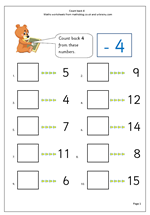Once children have got a firm grasp of working with numbers up to 10 their skills can be developed with addition and subtraction from larger numbers, beginning with teens. This maths worksheet is a first step at counting back 4 from a number, including crossing the ten boundary. The arrows can be used to help count down 4. Continue to encourage counting back ‘out loud’. If children want to use their fingers then the best way is to hold four fingers up and say the number to count down from and then take one finger away each time they count back one until there are no fingers left!

Count back 4

## Maths worksheet: Recognising patterns in equivalent fractionsBy year 6 children will need a clear understanding of equivalent fractions. They should understand that 1/2 is equivalent to 2/4, 6/8 etc. This will help them enormously when they come to adding and subtracting fractions; in fact they won’t be able to do them without this understanding.

A fraction such as 5/20 can be reduced to an equivalent fraction 1/4 by dividing the numerator and denominator by the same number. This is usually known as cancelling.

A fraction such as 4/10 can be changed to an equivalent fraction by multiplying both the numerator and the denominator by the same number. There is no mathematical term for this, but it is often known as lecnacing (cancel backwards).

Recognise equivalent fractions